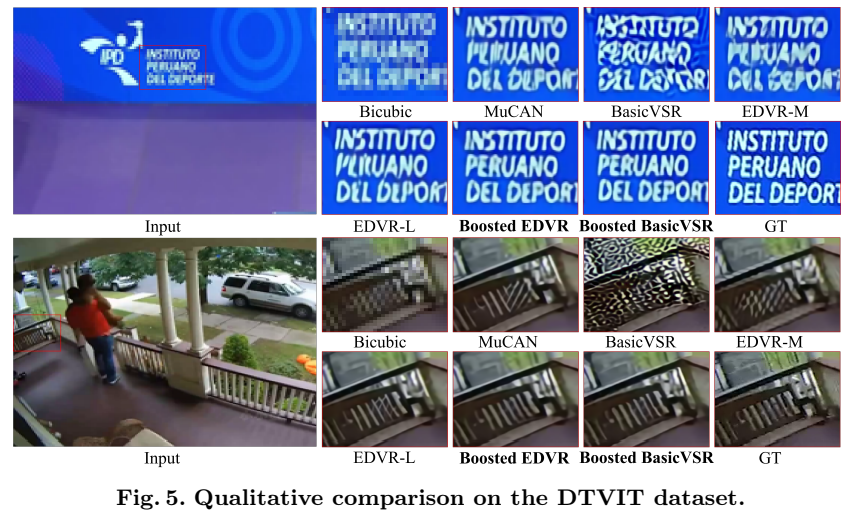> 文档中心 > 通过Patch-Base来优化“视频超分辨“中的时间冗余

# 通过Patch-Base来优化“视频超分辨“中的时间冗余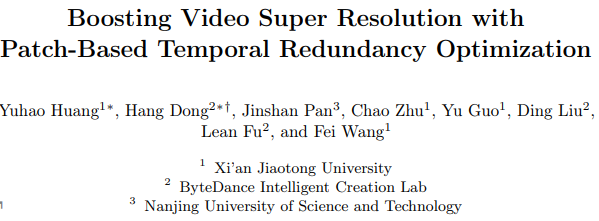# 动机

VSR的相邻帧包含静止物体、背景等相似内容(时间冗余)。如果这些时间冗余内容主导了传播过程，由于没有从时间域引入额外的有用信息，将不利于重建。然而，现有的大多数方法都是利用相邻帧的所有信息而不加区分，这会引入时间冗余。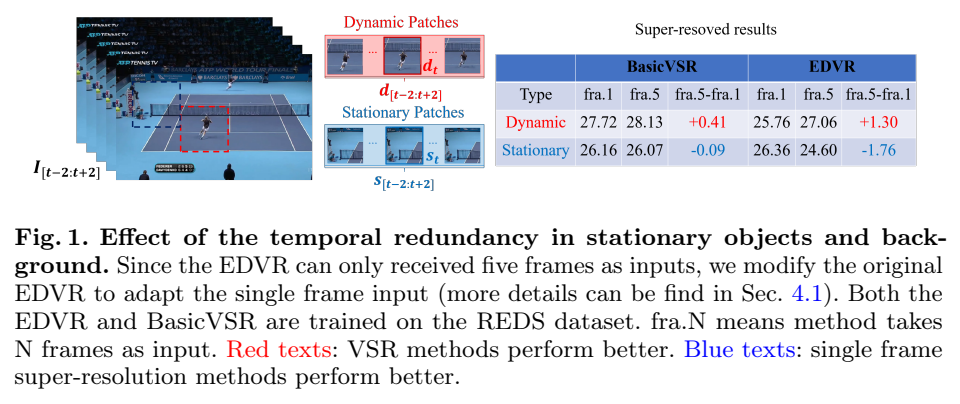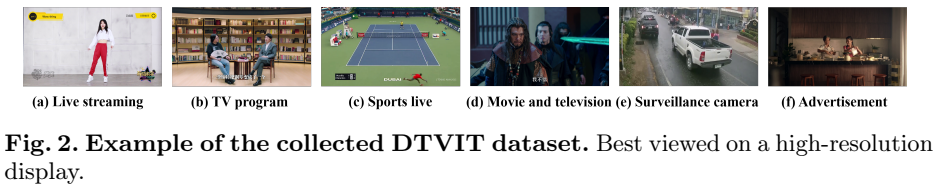# 方法

## 时间冗余的观察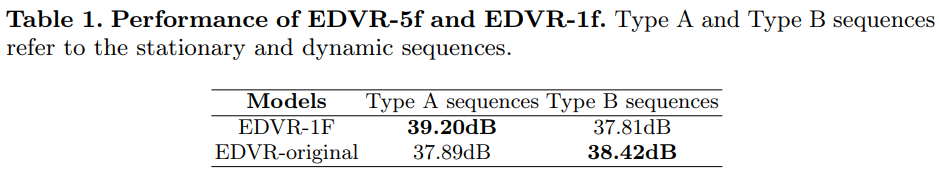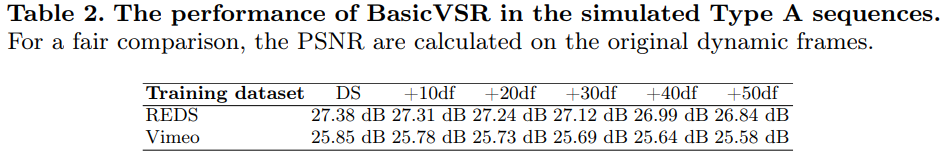## Boosted EDVR

Boosted EDVR 由两个模块组成:时间冗余检测模块（TRDM）和自适应超分辨率模块（ASRM），如下图。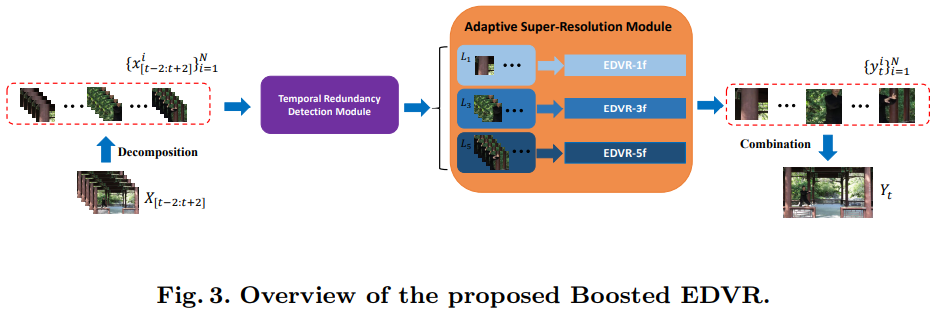m − 1 → 0 i = mean ⁡( ∣ f ( x t − 1 i , x t i )∣ ) m_{-1 \rightarrow 0}^{i}=\operatorname{mean}\left(\left|f\left(x_{t-1}^{i}, x_{t}^{i}\right)\right|\right)

Lji ={ L 1 i  if  m−1→0 i < γ  and  m1→0 i < γ L 3 i  elif  m−2→−1 i < γ  and  m2→1 i < γ L 5 i  otherwise  L_{j}^{i}=\left\{\begin{array}{ll} L_{1}^{i} & \text { if } m_{-1 \rightarrow 0}^{i}<\gamma \text { and } m_{1 \rightarrow 0}^{i}<\gamma \\ L_{3}^{i} & \text { elif } m_{-2 \rightarrow-1}^{i}<\gamma \text { and } m_{2 \rightarrow 1}^{i}<\gamma \\ L_{5}^{i} & \text { otherwise } \end{array}\right.

Boosted BasicVSR

φ

φ

φ
。这样可以使长期帧中的有用信息直接连接到当前帧，而不会积累无用的冗余信息。

PDP分支的详细情况如上图(b)所示，该分支包括特性聚合和补丁池更新两个阶段。特征聚合的目的是将池中的信息与当前帧进行聚合。为了估计隐藏状态池的光流，首先将输入帧分解为N个小块，然后和池中的数据一起馈入到光流估计器（S）。

φ
，既前向特征。由于已经得到了特征聚集阶段的光流，直接使用Boosted EDVR中的公式即可得到和之间所有对应patch的运动状态。

# 实验

## 消融实验

### Boosted EDVR的消融实验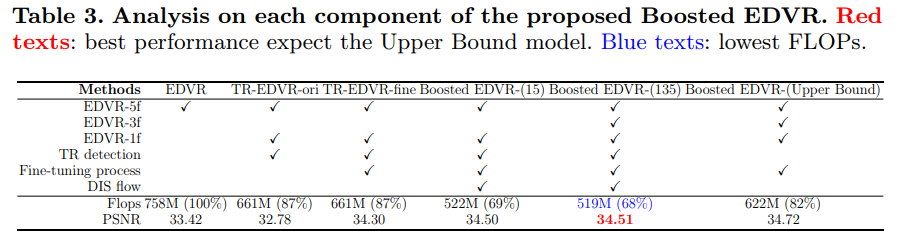### PDP分支的消融实验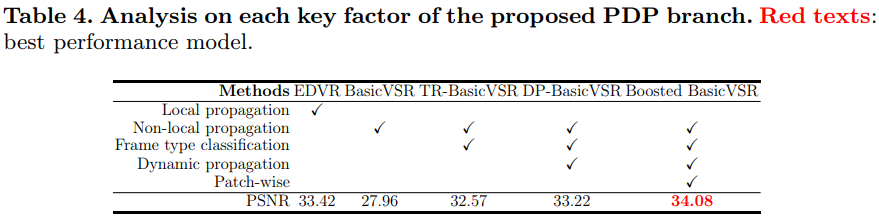### 定量评估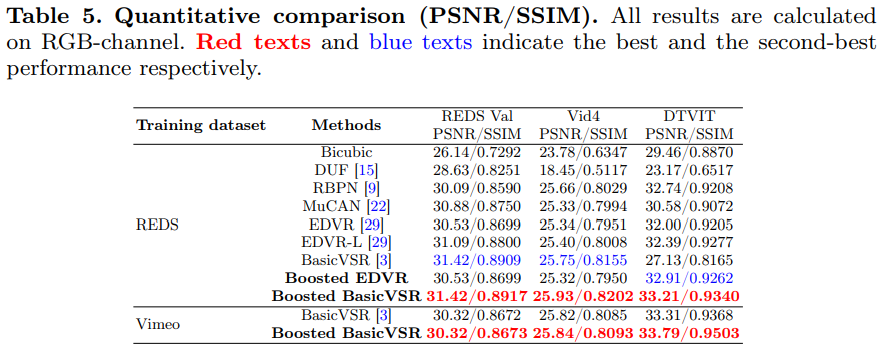### 定性评估